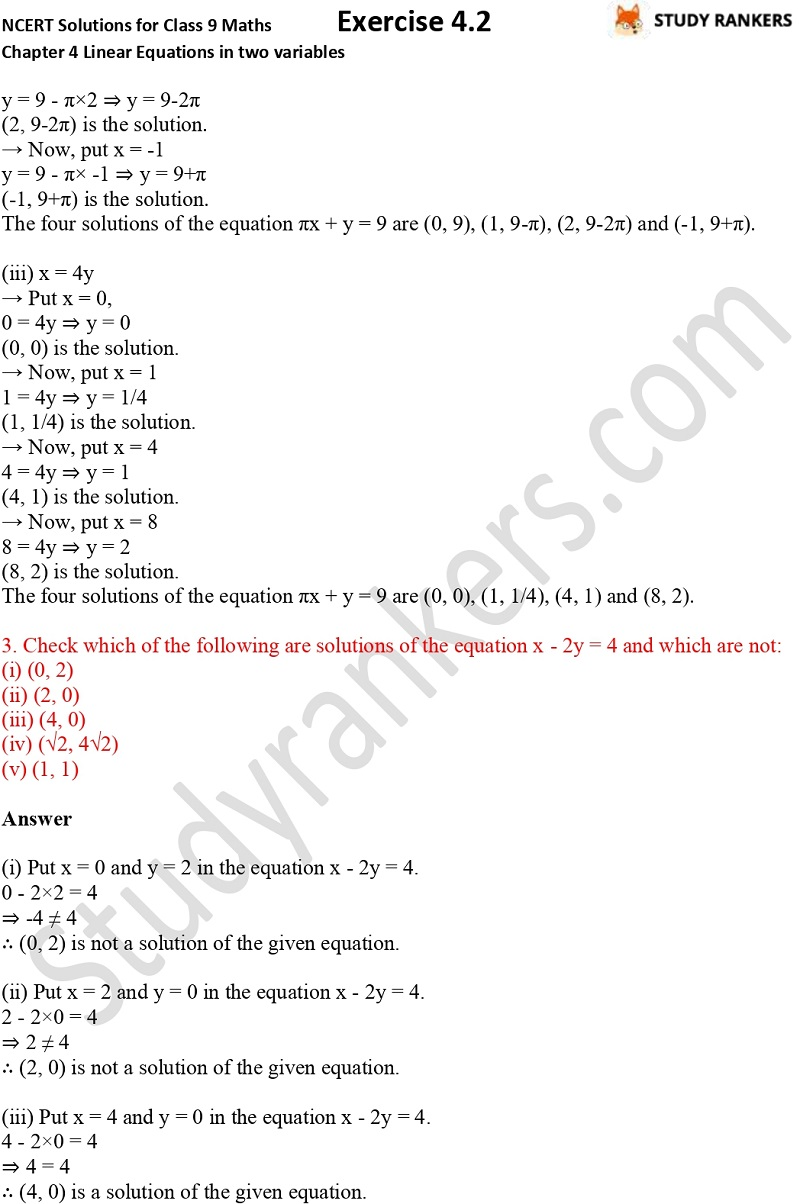## NCERT Solutions for Class 9 Maths Chapter 4 Linear Equations in Two Variables Exercise 4.2

Chapter 4 Linear Equations in Two Variables Exercise 4.2 NCERT Solutions for Class 9 Maths is useful in finding the solutions of difficult questions. Class 9 Maths NCERT Solutions will help you in finding important formulas and points so you can be aware of all the concepts of the chapter. These are prepared by subject matter experts which are detailed and accurate so you can complete your homework on time.

Ex 4.2 has total 4 questions which are about finding given options are true, and why, writing four solutions for each of the given equations etc.